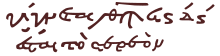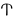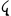# Greek numerals

﻿
Greek numerals
Numeral systems by culture
Hindu-Arabic numerals
Western Arabic (Hindu numerals)
Eastern Arabic
Indian family
Tamil
Burmese
Khmer
Lao
Mongolian
Thai
East Asian numerals
Chinese
Japanese
Suzhou
Korean
Vietnamese
Counting rods
Alphabetic numerals
Armenian
Āryabhaṭa
Cyrillic
Ge'ez
Greek (Ionian)
Hebrew
Other systems
Aegean
Attic
Babylonian
Brahmi
Egyptian
Etruscan
Inuit
Kharosthi
Mayan
Quipu
Roman
Sumerian
Urnfield
List of numeral system topics
Positional systems by base
Decimal (10)
2, 3, 4, 5, 6, 7, 8, 9, 11, 12, 13, 14, 15, 16, 20, 24, 30, 36, 60, 64
List of numeral systems
v · d · e
The numerical signs ʹ and ͵ redirect here. For the accent, ´, see Acute accent.

Greek numerals are a system of representing numbers using letters of the Greek alphabet. They are also known by the names Ionian numerals, Milesian numerals (from Miletus in Ionia), Alexandrian numerals, or alphabetic numerals (in common with other alphabetic numerations). In modern Greece, they are still used for ordinal numbers and in situations similar to those in which Roman numerals are still used elsewhere in the West. For ordinary cardinal numbers numbers, however, Greece uses Arabic numerals.

## History

Originally, before the adoption of the Greek alphabet, Linear A and Linear B had used a different system, called Aegean numbers, with symbols for 1, 10, 100, 1000 and 10000 operating with the following formula: | = 1, – = 10, ◦ = 100, ¤ = 1000, ☼ = 10000.

The earliest alphabet-related system of numerals used with the Greek letters was a set of the acrophonic Attic numerals, operating much like Roman numerals (which derived from this scheme), with: Ι = 1, Π = 5, Δ = 10, Η = 100, Χ = 1000, Μ = 10000; and with 50, 500, 5000, and 50000 represented by composites of Π and a tiny version of the applicable power of ten. The acrophonic system was replaced by a new alphabetic system, sometimes called the Ionic numeral system, from the 4th century BC.

## DescriptionGreek numerals in a Byzantine mathematical manuscript of Hero of Alexandria's Metrika, c.1100. The first line contains the number "͵θϡϟϛ δʹ ϛʹ", i.e. "9996 46". It features each of the special numeral symbols: sampi (ϡ,= 900), Koppa (ϟ,= 90, and two instances of digamma (ϛ,= 6), in their minuscule forms.

Each unit (1, 2, …, 9) was assigned a separate letter, each tens (10, 20, …, 90) a separate letter, and each hundreds (100, 200, …, 900) a separate letter. This requires 27 letters, so the 24-letter Greek alphabet was extended by using three obsolete letters: digamma ϝ for 6, qoppa ϟ for 90, and sampi ϡ for 900. Digamma is today most often written in its modern form ϛ, which is also called stigma; in Greece it is often replaced by the letter combination στ/ΣΤ. To distinguish numerals from letters they are followed in modern print by the keraia (Greek κεραία, "hornlike projection"), a symbol ( ʹ ) similar to an acute sign ( ´ ) but with its own Unicode character (U+0374). In ancient and medieval manuscripts, number signs were written with a horizontal line above.

This alphabetic system operates on the additive principle in which the numeric values of the letters are added together to form the total. For example, 241 is represented as σμαʹ (200 + 40 + 1). A famous example is 666 (the number of the Beast), which is represented as χξϛʹ (600 + 60 + 6) in medieval manuscripts of the Book of Revelation.

To represent numbers from 1,000 to 999,999 the same letters are reused to serve as thousands, tens of thousands, and hundreds of thousands. A "left keraia" (Unicode U+0375, ‘Greek Lower Numeral Sign’) is put in front of thousands to distinguish them from the standard use. For example, 2011 is represented as ͵βιαʹ (2000 + 11).

Letter Value Letter Value Letter Value
αʹ 1 ιʹ 10 ρʹ 100
βʹ 2 κʹ 20 σʹ 200
γʹ 3 λʹ 30 τʹ 300
δʹ 4 μʹ 40 υʹ 400
εʹ 5 νʹ 50 φʹ 500
ϝʹ or ϛʹ or στʹ 6 ξʹ 60 χʹ 600
ζʹ 7 οʹ 70 ψʹ 700
ηʹ 8 πʹ 80 ωʹ 800
θʹ 9 ϟʹ 90 ϡʹ 900

In modern Greek, uppercase letters are preferred, as in Φίλιππος Βʹ = Philip II.

## Higher numbers

To represent greater numbers, the Greeks also used the myriad from the old Attic numeral system in their notation. Its value is 10,000; the number of myriads was written above its symbol (Mʹ). For example (keraias replaced for technical reasons):$\stackrel{\text{,}\delta\phi\pi\beta}{\Mu}\psi\theta' = 4582\times 10,000+709 = 45,820,709. \,$

Other forms are also possible. When that didn't suffice the myriad myriad (one hundred million, symbol: ΜΜʹ) was used.

In his text The Sand Reckoner the natural philosopher Archimedes gives an upper bound of the number of grains of sand required to fill the entire universe, using a contemporary estimation of its size. This would defy the then-held notion that it is impossible to name a number greater than that of the sand on a beach, or on the entire world. In order to do that, he had to devise a new numeral scheme with much greater range.

## Hellenistic zero

Hellenistic astronomers extended alphabetic Greek numerals into a sexagesimal positional numbering system by limiting each position to a maximum value of 50 + 9 and including a special symbol for zero, which was also used alone like our modern zero, more than as a simple placeholder. However, the positions were usually limited to the fractional part of a number (called minutes, seconds, thirds, fourths, etc.) — they were not used for the integral part of a number. This system was probably adapted from Babylonian numerals by Hipparchus c. 140 BC. It was then used by Ptolemy (c. 140), Theon (c. 380), and Theon's daughter Hypatia (died 415).

In Ptolemy's table of chords, the first fairly extensive trigonometric table, there were 360 rows, portions of which looked as follows:$\begin{array}{ccc} \pi\varepsilon\varrho\iota\varphi\varepsilon\varrho\varepsilon\iota\tilde\omega\nu & \varepsilon\overset{\text{'}}\nu\vartheta\varepsilon\iota\tilde\omega\nu & \overset{\text{`}}\varepsilon\xi\eta\kappa\omicron\sigma\tau\tilde\omega\nu \\ \begin{array}{|l|} \hline \pi\delta\angle' \\ \pi\varepsilon \\ \pi\varepsilon\angle' \\ \hline \pi\stigma \\ \pi\stigma\angle' \\ \pi\zeta \\ \hline \end{array} & \begin{array}{|r|r|r|} \hline \pi & \mu\alpha & \gamma \\ \pi\alpha & \delta & \iota\varepsilon \\ \pi\alpha & \kappa\zeta & \kappa\beta \\ \hline \pi\alpha & \nu & \kappa\delta \\ \pi\beta & \iota\gamma & \iota\vartheta \\ \pi\beta & \lambda\stigma & \vartheta \\ \hline \end{array} & \begin{array}{|r|r|r|r|} \hline \circ & \circ & \mu\stigma & \kappa\varepsilon \\ \circ & \circ & \mu\stigma & \iota\delta \\ \circ & \circ & \mu\stigma & \gamma \\ \hline \circ & \circ & \mu\varepsilon & \nu\beta \\ \circ & \circ & \mu\varepsilon & \mu \\ \circ & \circ & \mu\varepsilon & \kappa\vartheta \\ \hline \end{array} \end{array}$

Each number in the first column, labeled περιφεριῶν, is the number of degrees of arc on a circle. Each number in the second column, labeled ενθειῶν, is the length of the corresponding chord of the circle, when the diameter is 120. Thus πδ represents an 84° arc, and the ∠' after it means one-half, so that πδ∠' means 84.5°. In the next column we see π μα γ, meaning 80 + 41/60 + 3/602. That is the length of the chord corresponding to an arc of 84.5° when the diameter of the circle is 120. The next column, labeled ὲξηκοστῶν, for "sixtieths", is the number to be added to the chord length for each 1° increase in the arc, over the span of the next 12°. Thus that last column was used for linear interpolation.

The Greek sexagesimal place holder or zero symbol changed over time. The symbol used on papyri during the second century was a very small circle with an overbar several diameters long, terminated or not at both ends in various ways. Later, the overbar shortened to only one diameter, similar to our modern o macron (ō) which was still being used in late medieval Arabic manuscripts whenever alphabetic numerals were used. But the overbar was omitted in Byzantine manuscripts, leaving a bare ο (omicron). This gradual change from an invented symbol to ο does not support the hypothesis that the latter was the initial of ουδέν meaning "nothing".

Some of Ptolemy's true zeros appeared in the first line of each of his eclipse tables, where they were a measure of the angular separation between the center of the Moon and either the center of the Sun (for solar eclipses) or the center of Earth's shadow (for lunar eclipses). All of these zeros took the form 0 | 0 0, where Ptolemy actually used three of the symbols described in the previous paragraph. The vertical bar (|) indicates that the integral part on the left was in a separate column labeled in the headings of his tables as digits (of five arc-minutes each), whereas the fractional part was in the next column labeled minute of immersion, meaning sixtieths (and thirty-six-hundredths) of a digit.